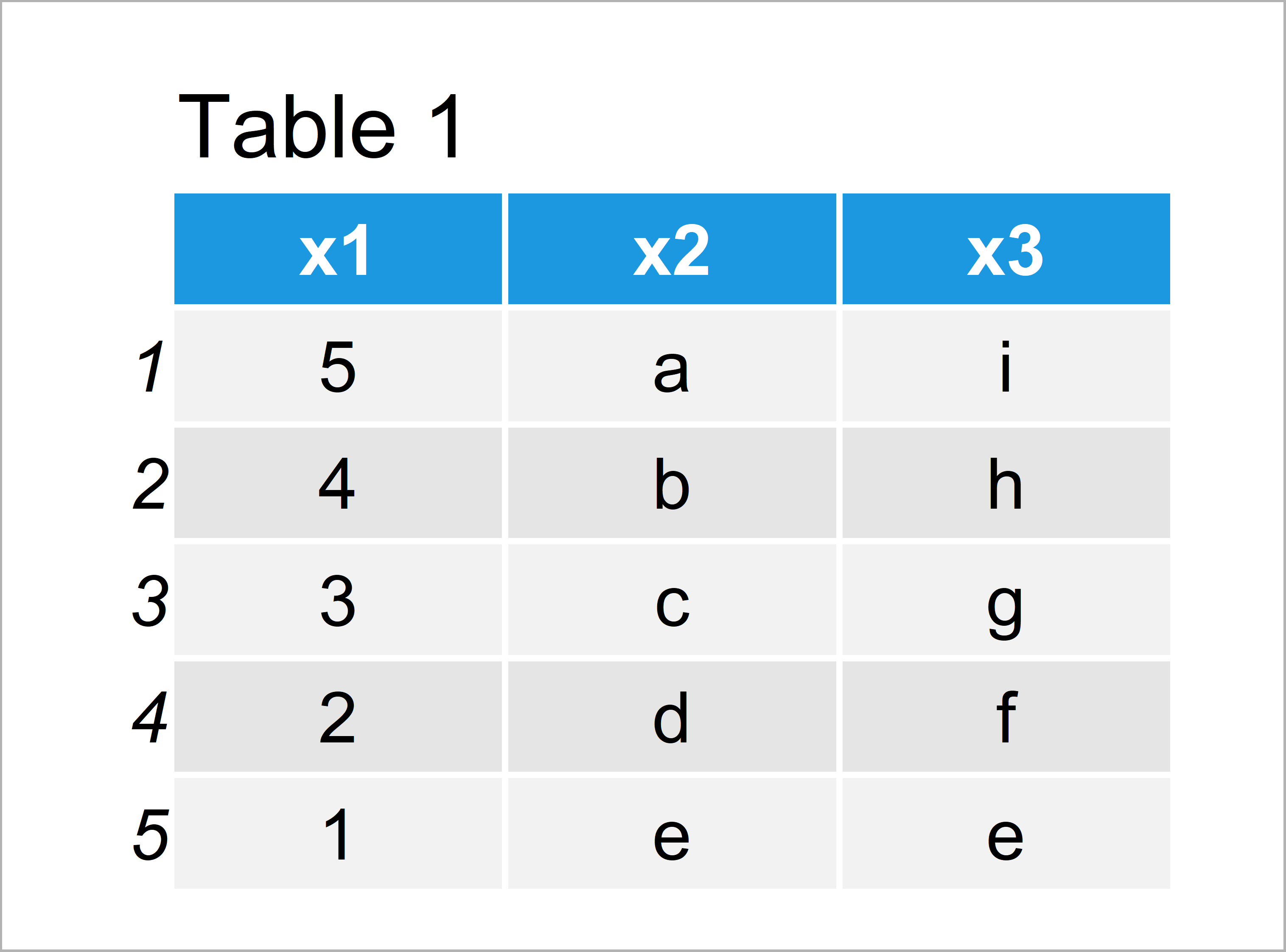# stringsAsFactors Argument of data frame Function in R (2 Examples)

In this tutorial, I’ll explain how to apply the stringsAsFactors argument of the data.frame function in R programming.

Let’s start right away.

## Example 1: Keep Character Class of Columns when Creating a Data Frame

In Example 1, I’ll explain how to keep the character class for variables of a data frame when creating a new data frame in R.

In this case, we have to specify the stringsAsFactors function to be equal to FALSE as shown in the following R code:

```data1 <- data.frame(x1 = 5:1,    # Specifying stringsAsFactors = FALSE
x2 = letters[1:5],
x3 = letters[9:5],
stringsAsFactors = FALSE)
data1                            # Print data frame```The output of the previous code is shown in Table 1 – We have created a data frame with three columns.

Let’s apply the class() function to check the data types of our variables:

```sapply(data1, class)             # Check classes of data frame columns
#          x1          x2          x3
#   "integer" "character" "character"```

The previous R code shows the class of each column, i.e. integer, character, and character.

Please note that stringsAsFactors = FALSE is the default specification of the data.frame function, in case you are using R version 4.0 or newer. In older versions, the default specification was stringsAsFactors = TRUE.

This is also explained in the help documentation of the data.frame function:

stringsAsFactors

logical: should character vectors be converted to factors? The ‘factory-fresh’ default has been TRUE previously but has been changed to FALSE for R 4.0.0. Only as short time workaround, you can revert by setting options(stringsAsFactors = TRUE) which now warns about its deprecation.

Anyway, let’s see what else we can do with the stringsAsFactors argument…

## Example 2: Convert Character Columns to Factors when Creating a Data Frame

The following R programming code explains how to automatically convert characters to factors when creating a new data frame.

For this, we simply need to specify the logical value TRUE to the stringsAsFactors argument:

```data2 <- data.frame(x1 = 5:1,    # Specifying stringsAsFactors = TRUE
x2 = letters[1:5],
x3 = letters[9:5],
stringsAsFactors = TRUE)
data2                            # Print data frame```In Table 2 it is shown that we have created another data frame by executing the previous R programming code.

The values of this data frame are exactly the same as in the previous example. However, the difference between the two data frames is shown by testing the classes of our new data frame columns:

```sapply(data2, class)             # Check classes of data frame columns
#        x1        x2        x3
# "integer"  "factor"  "factor"```

The variables x2 and x3 have been character strings in the previous example. In this example, however, they have the factor class.

## Video, Further Resources & Summary

In case you need further information on the examples of this article, you may watch the following video of my YouTube channel. I illustrate the contents of this article in the video.

In addition, you might want to have a look at the related tutorials of https://www.statisticsglobe.com/:

This tutorial has explained how to keep character classes when using the data.frame function in the R programming language.

By the way, the stringsAsFactors argument can also be used when importing data into R, e.g. when using the read.table or read.csv functions. However, the basic principles shown in this article can also be applied in this context.

Don’t hesitate to tell me about it in the comments section, in case you have additional questions.

Subscribe to the Statistics Globe Newsletter

•Melissa
December 31, 2022 7:00 pm

I am new to R. What does changing the data type to a factor do? Why would i do this? Why would i not do this?

•January 2, 2023 9:07 am